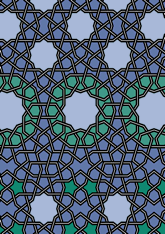data192/TRA0718

## Geometry

• The symmetry group of the tiling is 2*22 (cmm).
• All the internal angles of the constituent polygons are a multiple of 18°.
• Contains 14 regular pentagons.
• Contains two regular five-pointed star polygons with vertex angle of 72°.
• Contains four regular 10-pointed star polygons with vertex angle of 108°.
• There are 8 non-regular reflective tiles (including one kite).
• The tiling satisfies the interlace condition and has four finite interlaces and two infinite interlaces with straight cross-overs.
• The tiling is edge-to-edge.
• As drawn, contains about 473 polygons.

## References

Publications referenced: